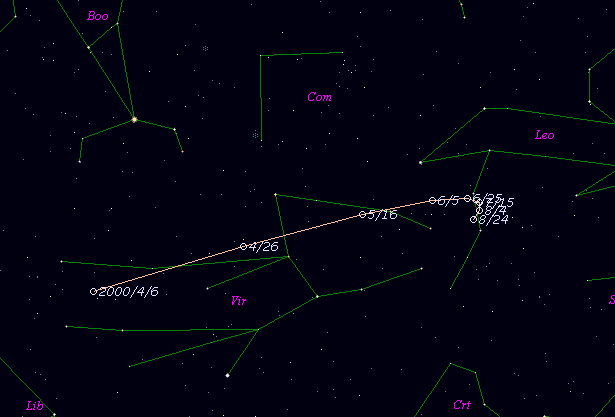# \$B%j%K%"WB@1(B

C/2000 G2 ( LINEAR )###\$B%W%m%U%#!<%k(B

 \$BH/8+F|(B 2000\$BG/(B4\$B7n(B4\$BF|(B \$BH/8+8wEY(B 18.4\$BEy(B \$BH/8+ Lincoln Laboratory Near-Earth Asteroid Research project

###\$B50F;MWAG(B

```   Improved orbital elements by K.Muraoka,
from 126 observations  2000 Apr. 4 to May 3,  m.e. = +/- 0".74.

T  =  2000 Feb.  6.1672  TT
Peri. =  101.7529
Node  =  328.3862   (2000.0)
Incl. =  170.4792
q  =    2.716787  AU
e  =    0.809221
a  =   14.240514  AU
n  =    0.0183407
P  =   53.74      years
```

###\$B@1?^(B###\$B8wEYJQ2=(B

```        m1 = 12.0 + 5 log\$B&\$(B + 10.0 log r
```##### \$B50F;MWAG\$OB<2,7r<#;a\$N7W;;\$K\$h\$k\$b\$N\$G\$9!#(B \$B@1?^\$O(B StellaNavigator Ver.2.0 for Windows (\$B%"%9%H%m%"!<%D(B \$BJTCx(B / \$B%"%9%-!<=PHG6I4)(B) \$B\$G:n@.\$7\$?\$b\$N\$G\$9!#(B \$B8wEY%0%i%U\$O(BComet for Windows\$B\$G:n@.\$7\$?\$b\$N\$G\$9!#(B